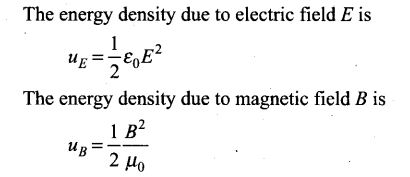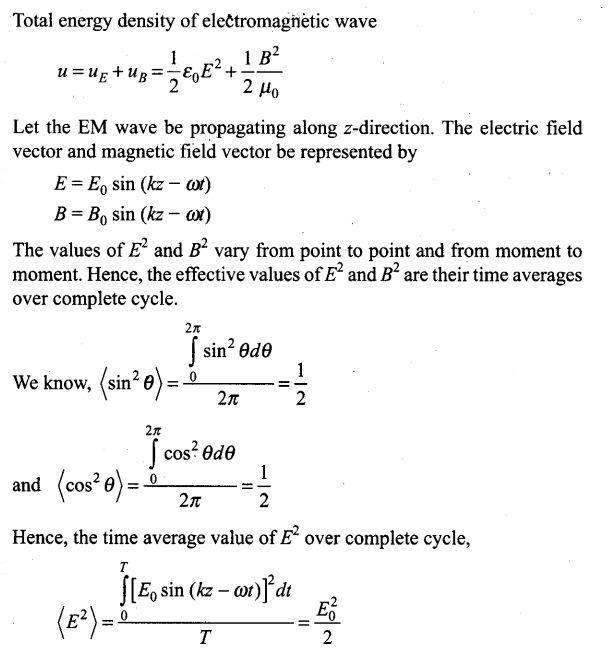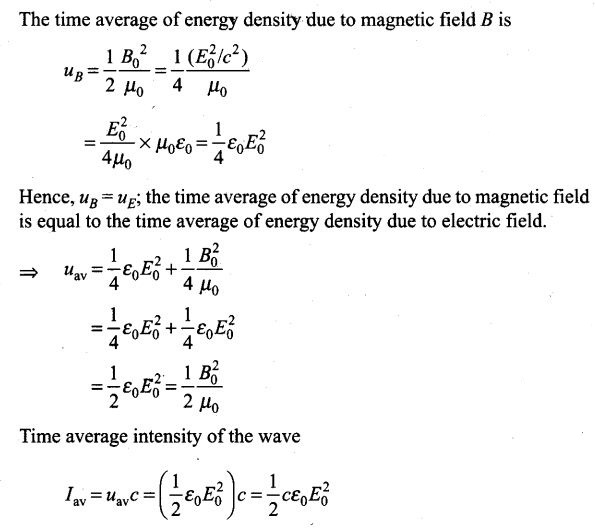# Electromagnetic Waves NCERT Exemplar Problems Solutions Physics

0
2469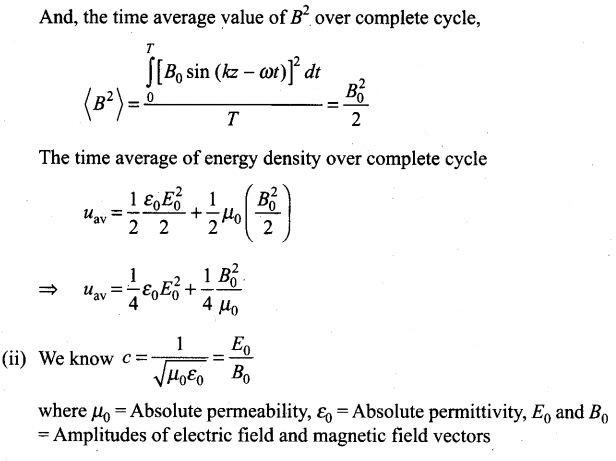## NCERT Exemplar Problems Class 12 Physics Chapter 8 Electromagnetic Waves

Multiple Choice Questions (MCQs)
Question 1. One requires 11 eV of energy to dissociate a carbon monoxide molecule into carbon and oxygen atoms. The minimum frequency of the appropriate electromagnetic radiation to achieve the dissociation lies in
(a) visible region (b) infrared region
(c) ultraviolet region (d) microwave region
Solution: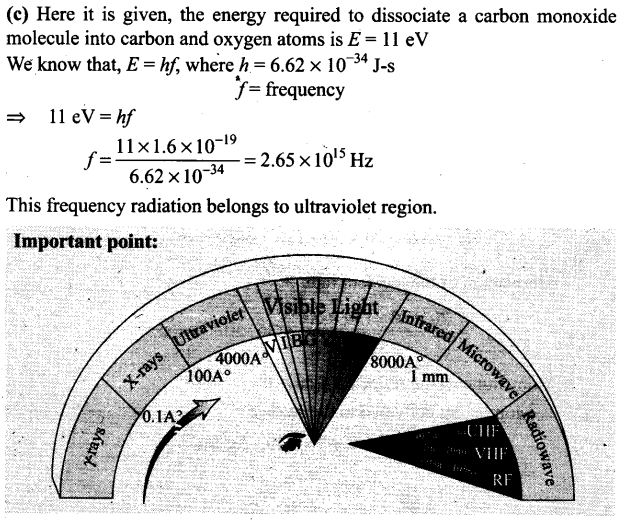Question 2.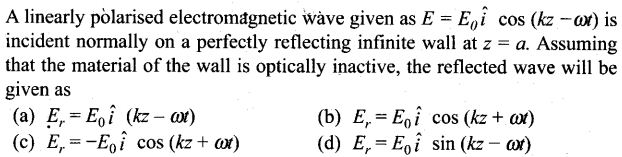Solution: (b)
Key concept: When a wave is reflected from a denser medium or perfectly reflecting wall made with optically inactive material, then the type of wave doesn’t change but only its phase changes by 180° or π radian.

Question 3. Light with an energy flux of 20 W/cm2 falls on a non-reflecting surface at normal incidence. If the surface has an area of 30 cm2, the total momentum delivered (for complete absorption) during 30 min is
(a) 36 x 10-5 kg-m/s (b) 36 x 10-4 kg-m/s
(c) 108 x 104 kg-m/s (d) 1.08 x 107 kg-m/s
Solution: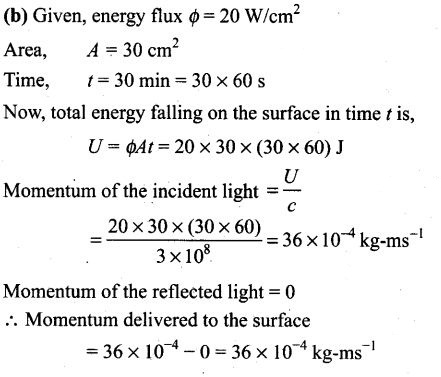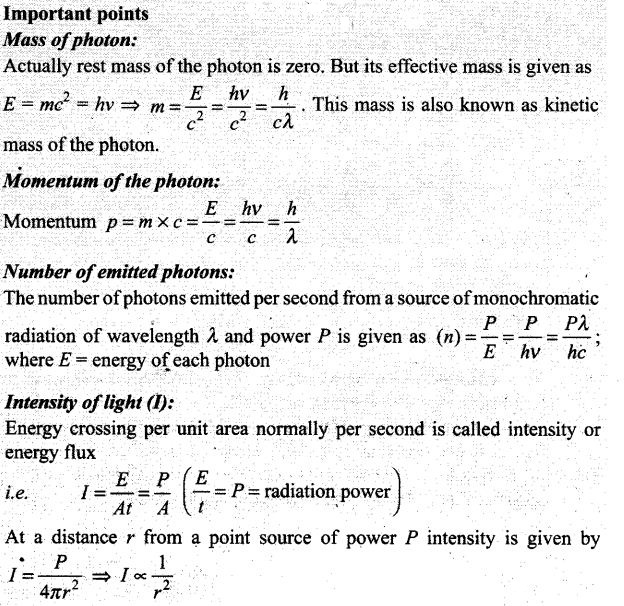Question 4. The electric field intensity produced by the radiations coming from a 100 W bulb at a 3 m distance is E. The electric field intensity produced by the radiations coming from 50 W bulb at the same distance is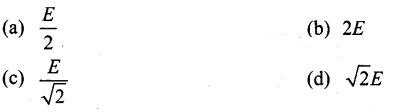Solution: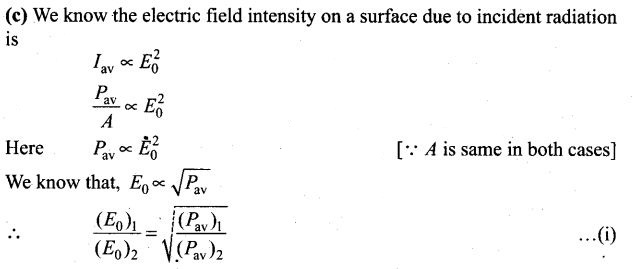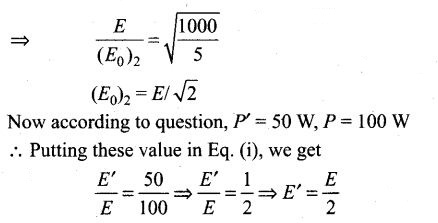Question 5.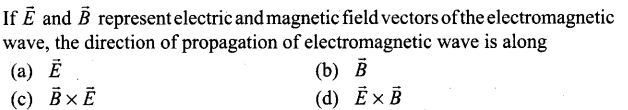Solution: (d)
Key concept: A changing electric field produces a changing magnetic field and vice versa which gives rise to a transverse wave known as electromagnetic wave. The time varying electric and magnetic field are mutually perpendicular to each other and also perpendicular to the direction of propagation of this wave. The electric vector is responsible for the optical effects of an EM wave and is called the light vector.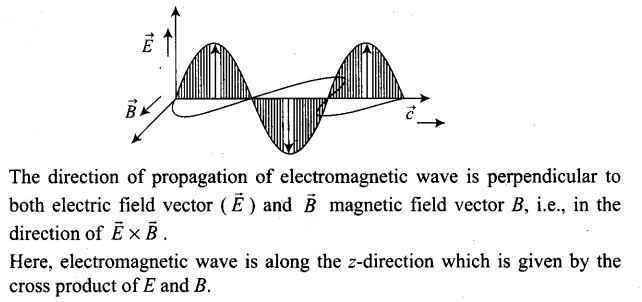Question 6. The ratio of contributions made by the electric field and magnetic field components to the intensity of an EM wave is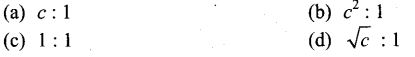Solution: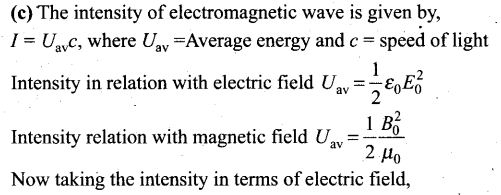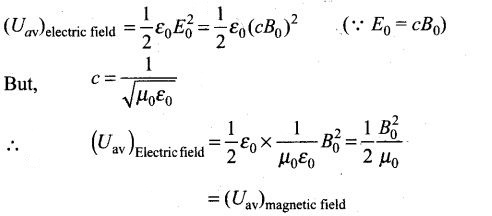Hence the energy in electromagnetic wave is divided equally between electric field vector and magnetic field vector.
It means the ratio of contributions by the electric field and magnetic field components to the intensity of an electromagnetic wave is 1:1.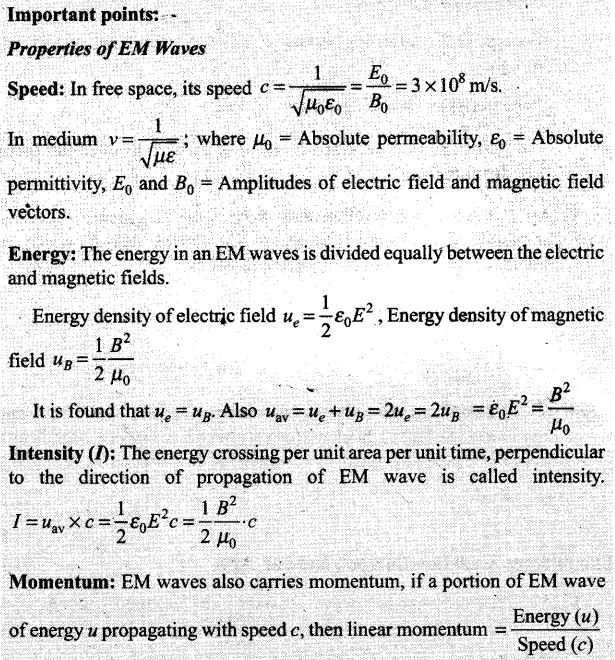• When the incident EM wave is completely absorbed by a surface, it delivers energy u and momentum u/c to the surface.
• When a wave of energy u is totally reflected from the surface, the momentum delivered to surface is 2u/c.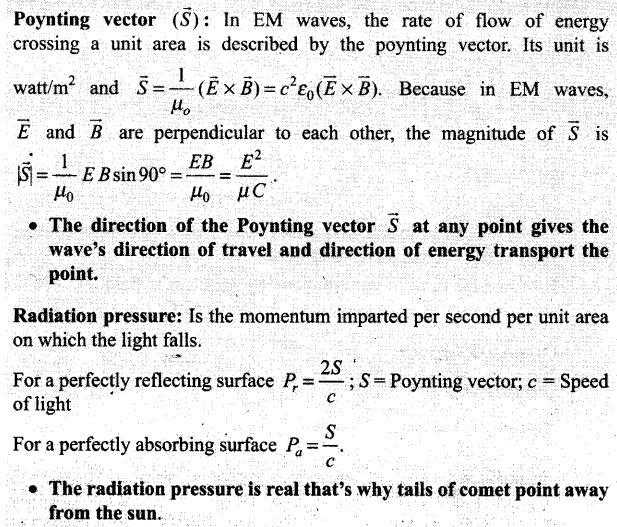Question 7. An EM wave radiates outwards from a dipole antenna, with E0 as the amplitude of its electric field vector. The electric field E0 which transports significant energy from the source falls off as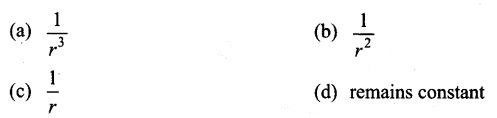Solution: (c) A diode antenna radiates the electromagnetic waves outwards. The amplitude of electric field vector (E0) which transports significant energy from the source falls intensity inversely as the distance (r) from the antenna,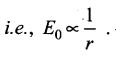One or More Than One Correct Answer Type
Question 8.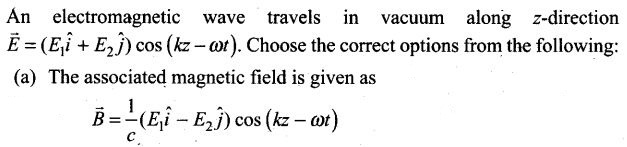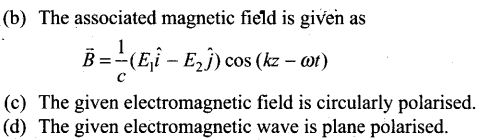Solution: (a, d) We are given that the electric field vector of an electromagnetic wave travels in a vacuum along z-direction as,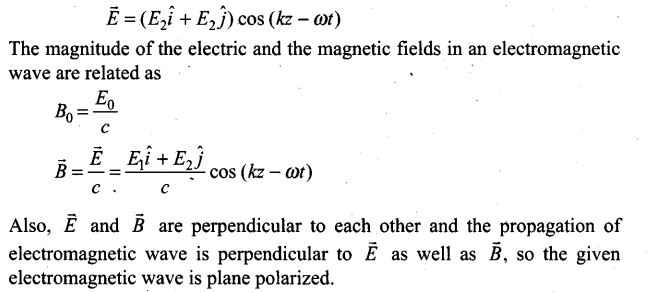Question 9.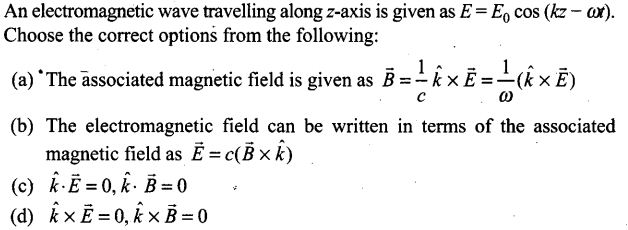Solution: (a, b, c)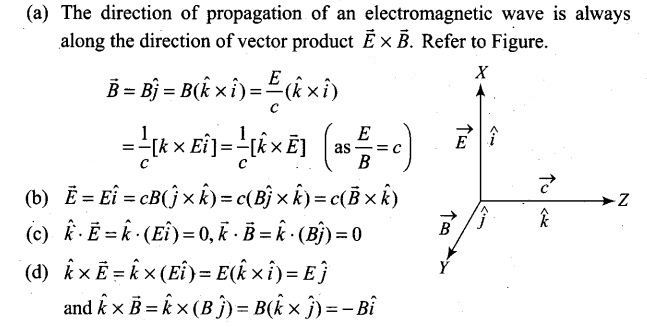Question 10. A plane electromagnetic wave propagating along x-direction can have the following pairs of E and B.
(a) Ex, By (b) Ey, Bz
(c) Bx,Ey (d) Ez,By
Solution: (b, d)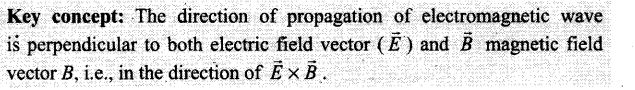Here in the question electromagnetic wave is propagating along x-direction. So, electro and magnetic field vectors should have either y-direction or 2-direction.

Question 11. A charged particle oscillates about its mean equilibrium position with a frequency of 109 Hz. The electromagnetic waves produced
(a) will have frequency of 109 Hz
(b) will have frequency of 2 x 109 Hz
(c) will have wavelength of 0.3 m
(d) fall in the region of radio waves
Solution: (a, c, d)
Here we are given the frequency by which the charged particles oscillates about its mean equilibrium position, it is equal to 109 Hz. The frequency of electromagnetic waves produced by a charged particle is equal to the frequency by which it oscillates about its mean equilibrium position.
So, frequency of electromagnetic waves produced by the charged particle is v= 109 Hz.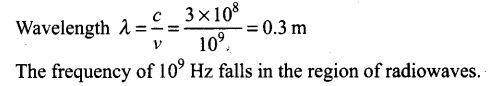Question 12. The source of electromagnetic waves can be a charge
(a) moving with a constant velocity
(b) moving in a circular orbit
(c) at rest
(d) falling in an electric field
Solution: (b, d)
Key concept:

• An electromagnetic wave can be produced by accelerated or oscillating charge.
• An oscillating charge is accelerating continuously, it will radiate electromagnetic waves continuously.
• Electromagnetic waves are also produced when fast moving electrons are suddenly stopped by a metal target of high atomic number.

Here, in option (b) charge is moving in a circular orbit.
In circular motion, the direction of the motion of charge is changing continuously, thus it is an accelerated motion and this option is correct.
In option (d), the charge is falling in electric field. If a charged particle is moving in electric field it experiences a force or we can say it accelerates. We know an accelerating charge particle radiates electromagnetic waves. Hence option (d) is also correct.
Also, we know that a charge starts accelerating when it falls in an electric field.
Important points:

• In an atom an electron is circulating around the nucleus in a stable orbit, although accelerating does not emit electromagnetic waves; it does so only when it jumps from a higher energy orbit to a lower energy orbit.
• A simple LC oscillator and energy source can produce waves of desired frequency

Question 13. An EM wave of intensity I falls on a surface kept in vacuum and exerts radiation pressure p on it. Which of the following are true?
(a) Radiation pressure is I/c if the wave is totally absorbed
(b) Radiation pressure is —I/c if the wave is totally reflected
(c) Radiation pressure is 2I/c if the wave is totally reflected
(d) Radiation pressure is in the range I/c < p < 2I/c for real surfaces
Solution: (a, c, d)
Key concept: Radiation pressure (p) is the force exerted by electromagnetic wave on unit area of the surface, i.e., rate of change of momentum per unit area of the surface.
Let us consider a surface exposed to electromagnetic radiation as shown in figure. The radiation is falling normally on the surface. Further, intensity of radiation is I and area of surface exposed to radiation is A.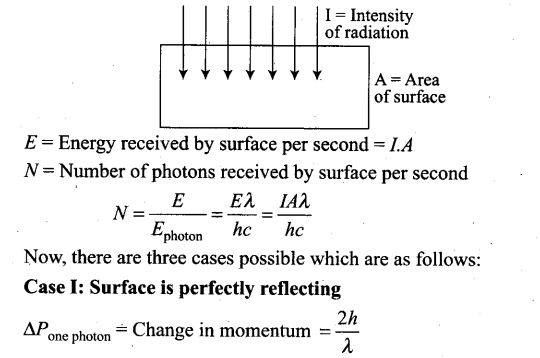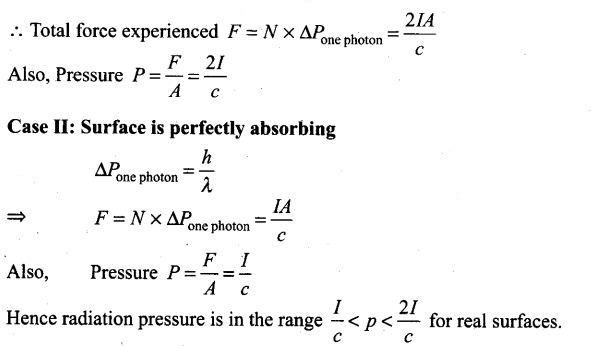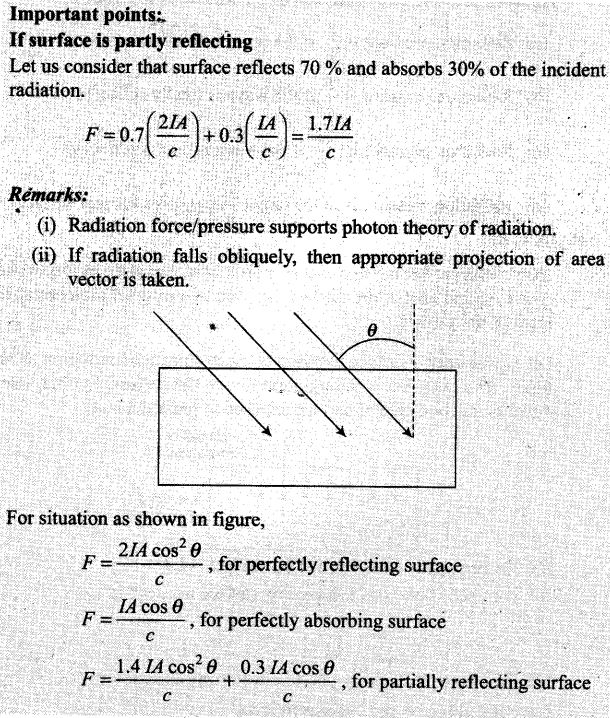Question 14. Why is the orientation of the portable radio with respect to broadcasting station important?
Solution: The electromagnetic waves are plane polarised, so the receiving antenna should be parallel to the vibration of the electric or magnetic field of the wave. So the receiving antenna should be parallel to electric/magnetic part of the wave. That is why the orientation of the portable radio with respect to broadcasting station is important.

Question 15. Why does microwave oven heats up a food item containing water molecules most efficiently?
Solution: The microwave oven heats up the food items containing water molecules most efficiently because the frequency of microwaves matches the resonant frequency of water molecules.

Question 16. The charge on a parallel plate capacitor varies as q=q0 cos 2πvt. The plates are very large and close together (area = A, separation = d). Neglecting the edge effects, find the displacement current through the capacitor.
Solution: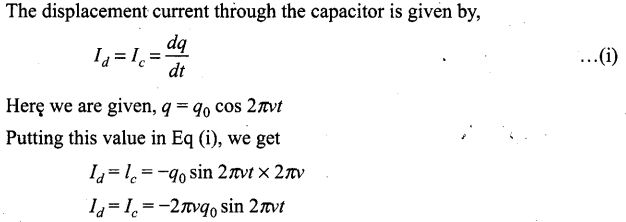Question 17. A variable frequency AC source is connected to a capacitor. How will the displacement current change with decrease in frequency?
Solution: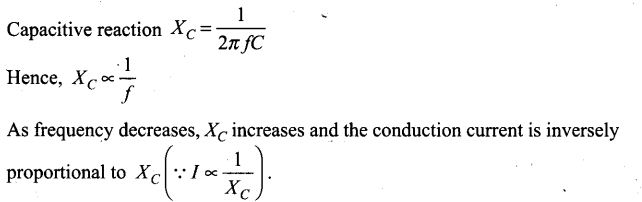It means the displacement current decreases as the conduction current is equal to the displacement current.

Question 18. The magnetic field of a beam emerging from a fitter facing a flood light is given by B0= 12 x 10-8 sin (1.20 x 107z- 3.60 x 1015 t) T What is the average intensity of the beam?
Solution: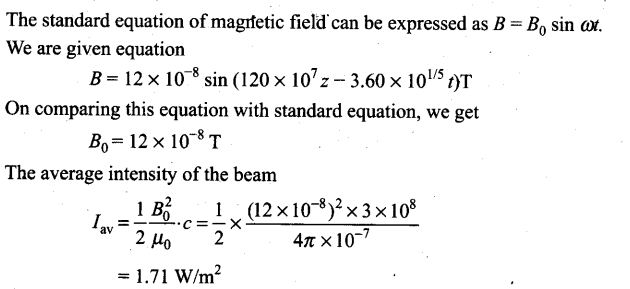Question 19.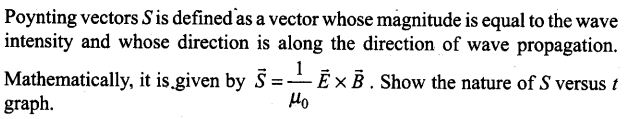Solution: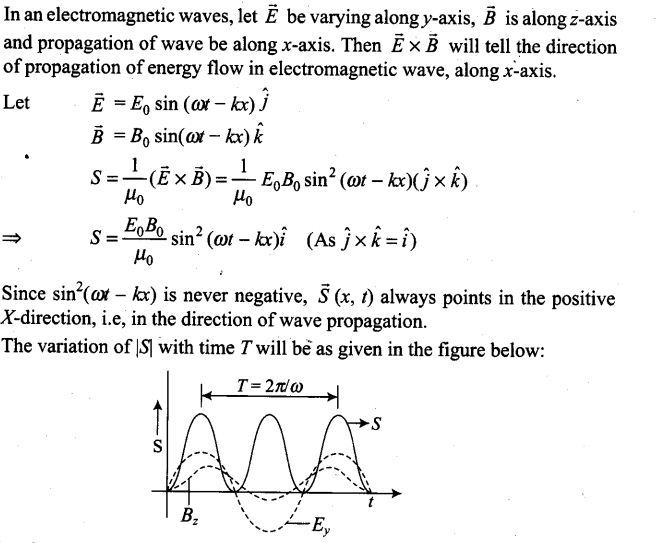Question 20. Professor CV Raman surprised his students by suspending freely a tiny light ball in a transparent vacuum chamber by shining a laser beam on it. Which property of EM waves was he exhibiting? Give one more example of this property.
Solution: The properties of an electromagnetic wave is same as other waves. Like other wave an electromagnetic wave also carries energy and momentum. Since, it carries momentum, an electromagnetic wave also exerts pressure called radiation pressure. This property of electromagnetic waves helped professor C V Raman surprised his students by suspending freely a tiny light ball in a transparent vacuum chamber by shining a laser beam on it.

Question 21.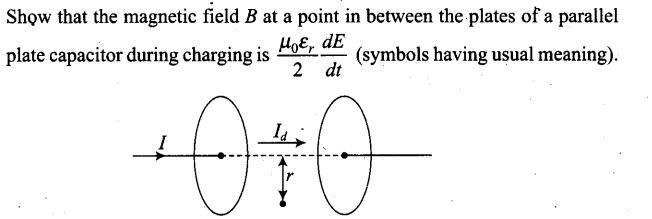Solution: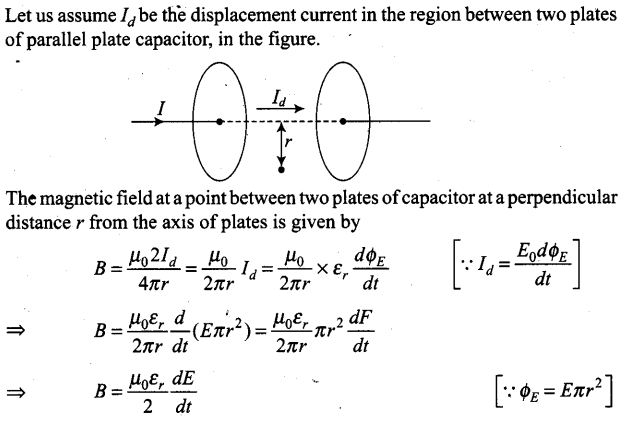Question 22. Electromagnetic waves with wavelength
(i) λ1, is used in satellite communication.
(ii) λ2, is used to kill germs in water purifier.
(iii) λ3, is used to detect leakage of oil in underground pipelines.
(iv) λ4, is used to improve visibility in runways during fog and mist conditions.
(a) Identify and name the part of electromagnetic spectrum to which these radiations belong.
(b) Arrange these wavelengths in ascending order of their magnitude.
(c) Write one more application of each.
Solution: (a) (i) In satellite communications, microwave is widely used. Hence λ1, is the wavelength of microwave.
(ii) In water purifier, ultraviolet rays are used to kill germs. So, λ2 is the wavelength of UV rays.
(iii) X-rays are used to detect leakage of oil in underground pipelines. So, λ3 is the wavelength of X-rays.
(iv) Infrared rays are used to improve visibility on runways during fog and mist conditions. So, it is the wavelength of infrared waves.
(b) Wavelength of X-rays < wavelength of UV < wavelength of infrared < wavelength of microwave.
=> λ3241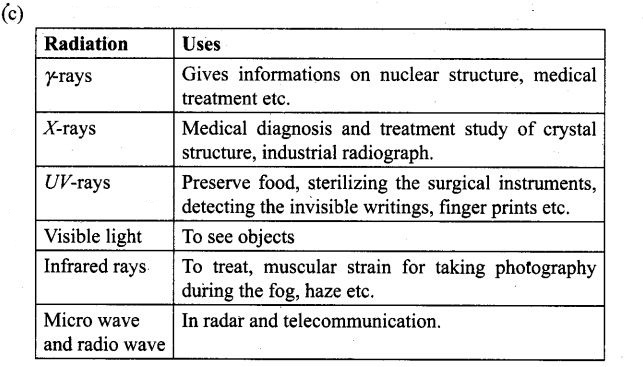Question 23.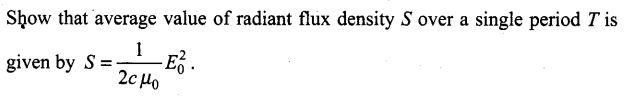Solution: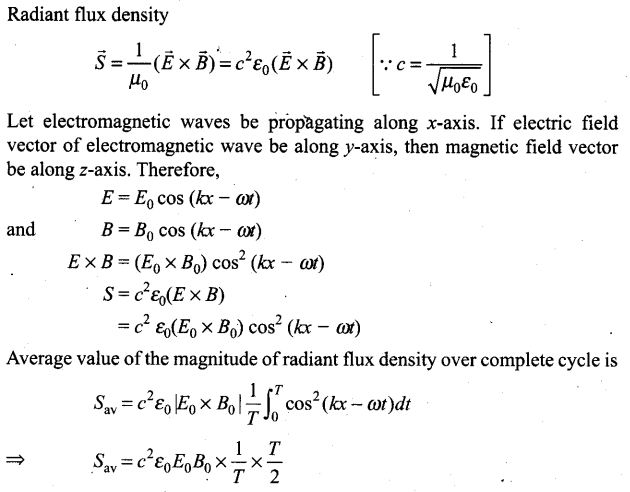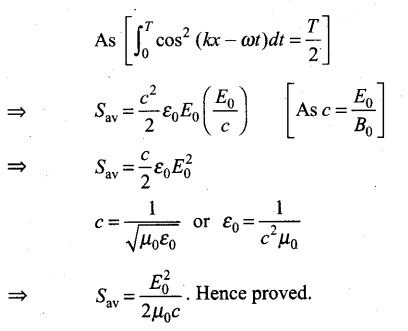Question 24. You are given a 2 μF parallel plate capacitor. How would you establish an instantaneous displacement current of 1 mA in the space between its plates?
Solution: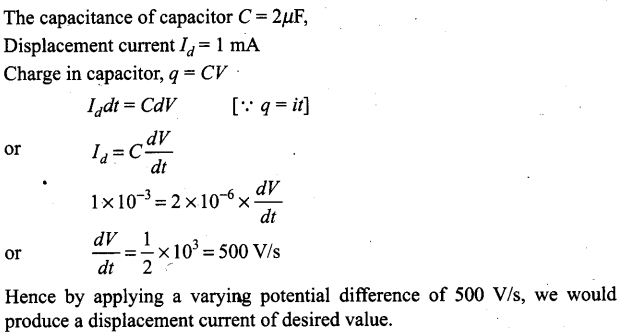Question 25. Show that the radiation pressure exerted by an EM wave of intensity I on a surface kept in vacuum is I/C.
Solution: Let us consider a surface exposed to electromagnetic radiation. The radiation is falling normally on the surface. Further, intensity of radiation is I and area of surface exposed to radiation is A.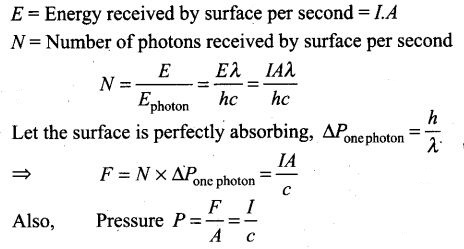Question 26. What happens to the intensity of light from a bulb if the distance from the bulb is doubled? As a laser beam travels across the length of room, its intensity essentially remains constant.
What geometrical characteristic of LASER beam is responsible for the constant intensity which is missing in the case of light from the bulb?
Solution: We know intensity of light from a point source I α 1/r2, r is the distance from point source.
As the distance is doubled, so the intensity becomes one-fourth the initial value. But in case of laser it does not spread, so its intensity remain same.
Some geometrical characteristics of LASER beam which are responsible for the constant intensity is
(i) Unidirection (ii) Monochromatic
(iii) Coherent light (iv) Highly collimated
These characteristics are missing in the case of normal light from the bulb.

Question 27. Even though an electric field E exerts a force qE on a charged particle yet electric field of an EM wave does not contribute to the radiation pressure (but transfers energy). Explain.
Solution: The electric field of an electromagnetic wave is an oscillation field. It exerts electric force on a charged particle, but this electric force averaged over an integral number of cycles is zero, since its direction changes every half cycle. Hence, electric field is not responsible for radiation pressure though it transfer the energy. In fact, radiation pressure appears as a result of the action of the magnetic field of the wave on the electric currents induced by the electric field of the same wave.

Question 28.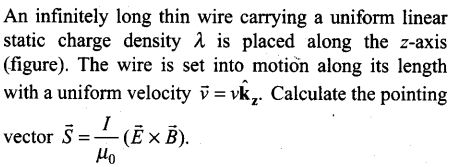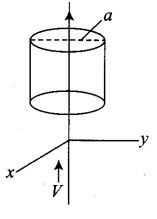Solution: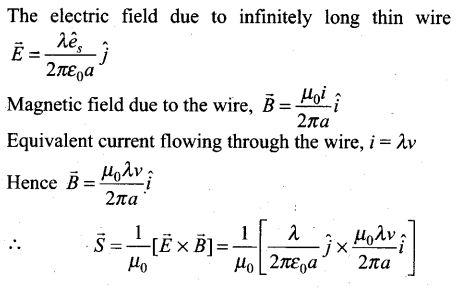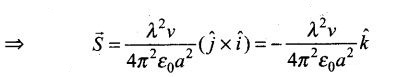Question 29.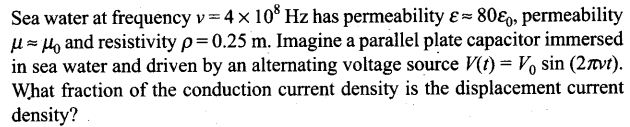Solution: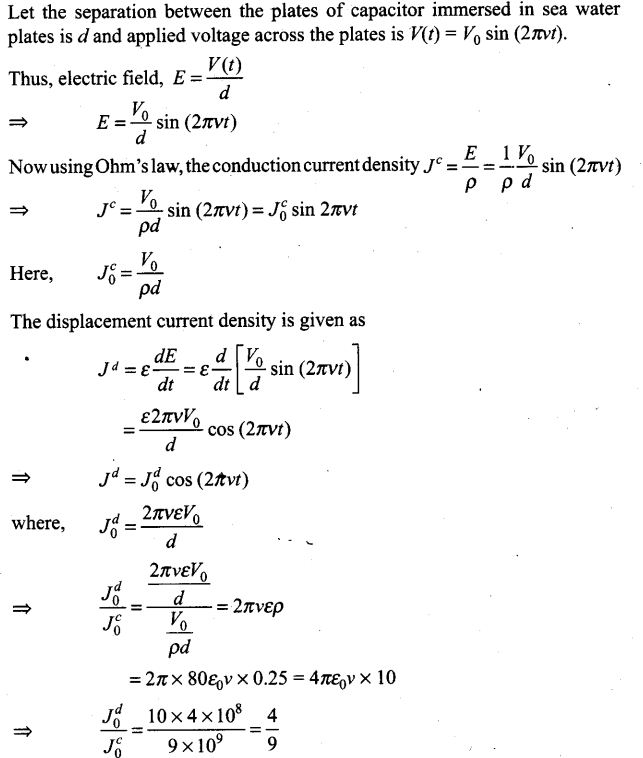Question 30.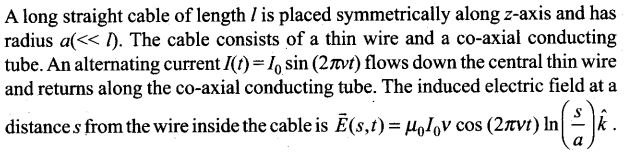(i) Calculate the displacement current density inside the cable.
(ii) Integrate the displacement current density across the cross-section of the cable to find the total displacement current ld.
(iii) Compare the conduction current I0 with the displacement current I0d.
Solution: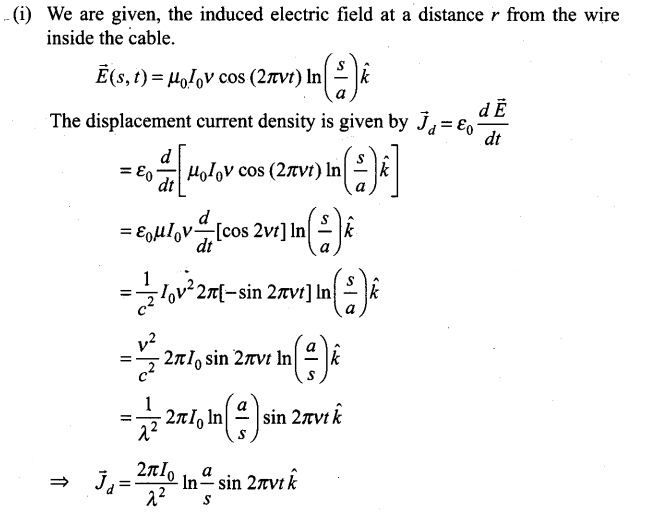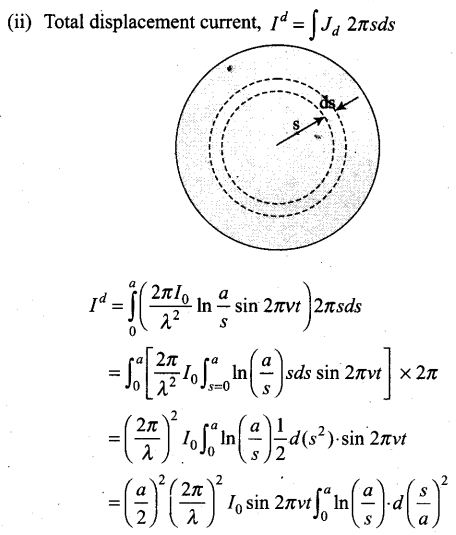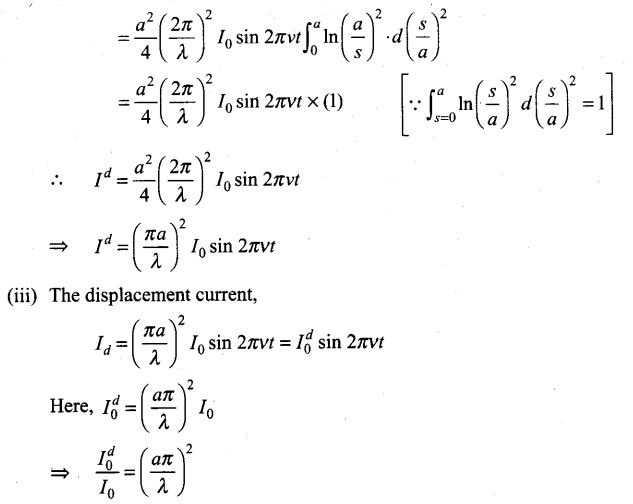Question 31.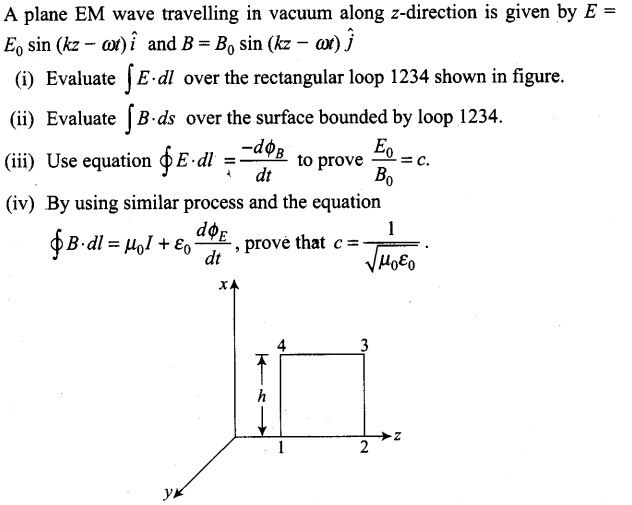Solution: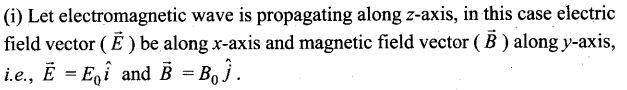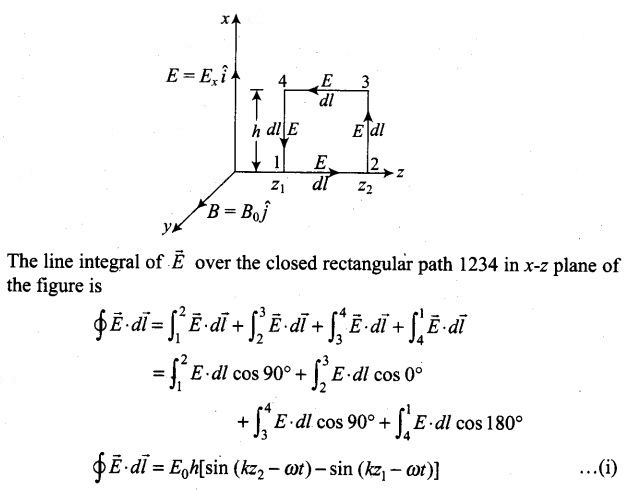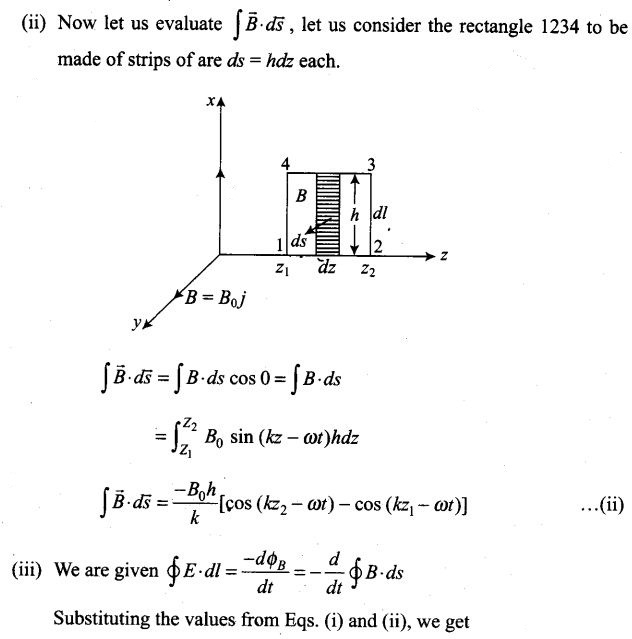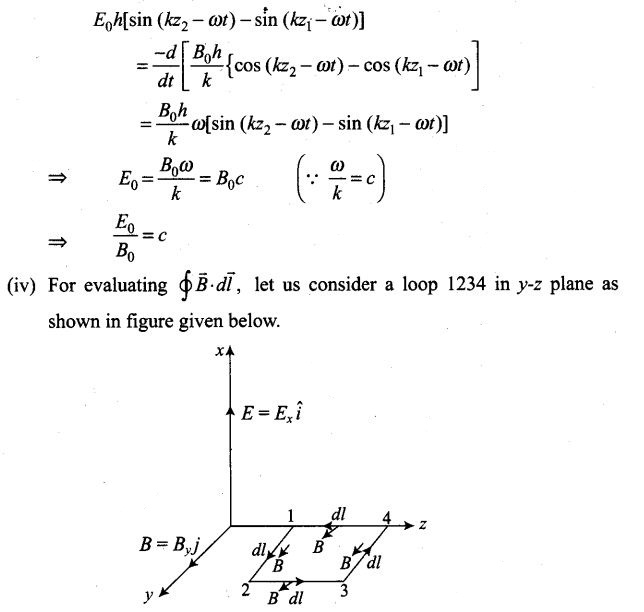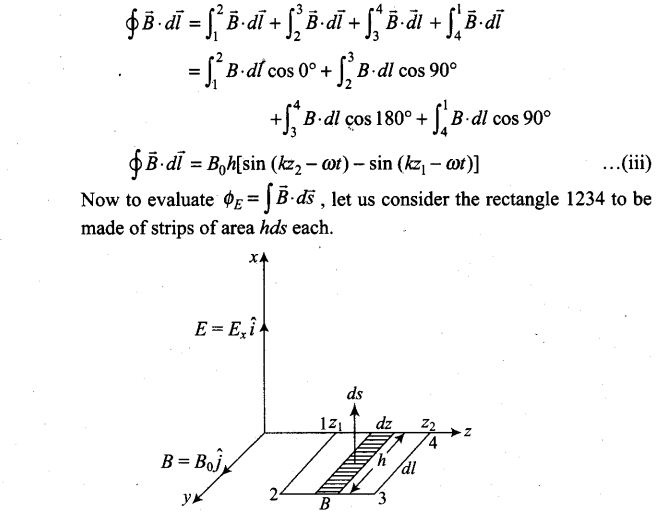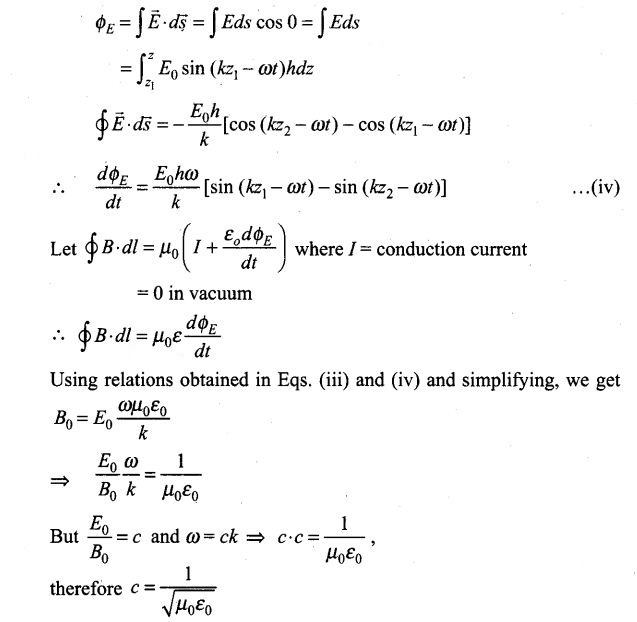Question 32.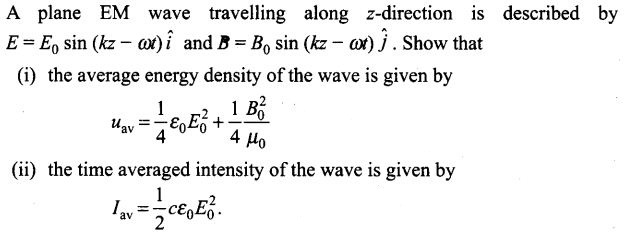Solution: (i) Total energy carried by electromagnetic wave is due to electric field vector and magnetic field vector. In electromagnetic wave, E and B vary from point to point and from moment to moment.